### HESSIAN Statement

HESSIAN variables ;

The HESSIAN statement defines the Hessian matrix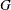containing the second-order derivatives of the objective function
with respect to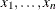. For more information, see the section Derivatives.

If the DIAHES option is not specified, the HESSIAN statement lists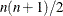variable names which correspond to the elements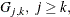of the lower triangle of the symmetric Hessian matrix listed by rows. For example, the statements

      min f;
decvar x1 - x3;
hessian g1-g6;


correspond to the Hessian matrix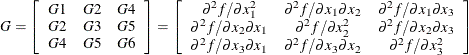If the DIAHES option is specified, only thediagonal elements must be listed in the HESSIAN statement. Therows andcolumns of the Hessian matrixmust correspond to the order of theparameter names listed in the DECVAR statement. To specify the values of nonzero derivatives, the variables specified in the HESSIAN statement must be defined on the left-hand side of algebraic expressions in the programming statements. For example, consider the Rosenbrock function:

proc nlp tech=nrridg;
min f;
decvar x1 x2;
hessian h1-h3;

f1 = 10 * (x2 - x1 * x1);
f2 = 1 - x1;

f = .5 * (f1 * f1 + f2 * f2);

g1 = -200 * x1 * (x2 - x1 * x1) - (1 - x1);
g2 = 100 * (x2 - x1 * x1);

h1 = -200 * (x2 - 3 * x1 * x1) + 1;
h2 = -200 * x1;
h3 = 100;
run;